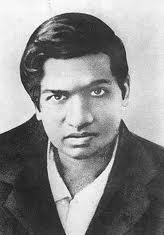# Ramanujan’s Taxicab NumberMathematical discoveries are not always birthed in the delivery room of revolutionary thinking.  Often times, they are found in small interactions that emerge from casual conversations.  Throughout history, the frontiers of mathematics have been riddled with concepts protruding from the foundation of humble beginnings.    With this in mind, many mathematicians see collaboration, both small and large, as an important key to advancing their respective fields.

This can readily be seen in the early part of the twentieth century.    In 1914, the prodigious mathematician, Srinivasa Ramanujan, left his native home in Madras, India and traveled to the University of Cambridge in England at the invitation of two legendary mathematicians, G.H. Hardy and J.E. Littlewood.    A year prior to his arrival, Ramanujan sent a letter to Hardy that contained a collage of mathematical notation scattered throughout the text.    At first glance, Hardy dismissed the letter as gibberish.

However, after a more careful examination from both Hardy and Littlewood, they came to the conclusion that it was the work of a genius.   This started an ongoing collaboration that yielded some of the most elegant work ever produced in the history of mathematics.  In light of this,  it could be said that Ramanujan’s time in England was bittersweet.   While living in Cambridge, he became ill due to the contrasting climates between England and India.  Hardy later retold a story about visiting Ramanujan during his illness:

“I remember once going to see him when he was lying ill at Putney. I had ridden in taxi cab number 1729 and remarked that the number seemed to me rather a dull one, and that I hoped it was not an unfavorable omen. ‘No,’ he replied, ‘it is a very interesting number; it is the smallest number expressible as the sum of two cubes in two different ways.’ “

As Ramanujan pointed out, 1729 is the smallest number to meet such conditions.  More formally,$1729 = 1^{3} +12^{3}$ and$1729 = 9^{3} + 10^{3}$.  In honor of the Ramanujan-Hardy conversation, the smallest number expressible as the sum of two cubes in$n$ different ways is known as the$nth$ taxicab number and is denoted as$Taxicab (n)$ .  Therefore, with this notation, we see that$Taxicab(2) = 1729$.

Extending this concept a little further, a generalized taxicab number can be defined as the smallest number that can be expressed as a sum of a$j$ number of$kth$ powers in $n$ different ways and is denoted as$Taxicab(k,j,n)$.  For example,$Taxicab(4,2,2) = 635318657$, since$635318657= 59^{4} + 158^{4}$ ,$635318657= 133^{4} + 134^{4}$, and$635318657$ is the smallest such number that meets the parameters given by$k = 4$ ,$j=2$, and$n=2$.

Interestingly enough, no one knows what the general taxicab number,$Taxicab(5,2,n)$,  equals for any$n > 1$.   Even for the weak version, a solution has not been provided.  In other words, if one removes the condition that the number has to be the smallest and we let$n=2$, the question can be restated the following way:  Does there exist any number that is expressible as the sum of two positive fifth powers in two different ways?

So far, all attempts to prove the weaker version have failed.  One possible attack is to produce an example computationally.   Another method would be to prove or disprove its existence rigorously.  In any case, don’t underestimate the effectiveness of good collaboration emerging from casual conversations.## About Avery Carr

Avery Carr is a senior analyst and past senior editor for the American Mathematical Society Grad Blog. He and his wife, Alison, live in Olive Branch, MS.
This entry was posted in AMS, Math, Mathematics in Society, Mathematics Online, Uncategorized. Bookmark the permalink.